In a regular quadrilateral pyramid, the side edge is e = 7 dm and the diagonal of the base is 50 cm. Calculate the pyramid shell area.

Correct result:

S =  47.8931 dm2

#### Solution: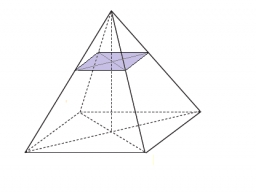We would be pleased if you find an error in the word problem, spelling mistakes, or inaccuracies and send it to us. Thank you!Tips to related online calculators

#### You need to know the following knowledge to solve this word math problem:

We encourage you to watch this tutorial video on this math problem:

## Next similar math problems:

• Ten persons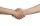Ten persons, each person makes a hand to each person. How many hands were given?
• Each with each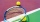Five pupils from 3A class played table tennis. How many matches did they play with each other?
• MO Z8–I–6 2018In the KLMN trapeze, KL has a 40 cm base and an MN of 16 cm. Point P lies on the KL line so that the NP segment divides the trapezoid into two parts with the same area. Find the length of the KP line.
• Diagonals of pentagonCalculate the diagonal length of the regular pentagon: a) inscribed in a circle of radius 12dm; b) a circumscribed circle with a radius of 12dm.
• Diagonals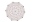What x-gon has 54 diagonals?
• Hexagon rotationA regular hexagon of side 6 cm is rotated through 60° along a line passing through its longest diagonal. What is the volume of the figure thus generated?
• Diagonals in diamondIn the rhombus is given a = 160 cm, alpha = 60 degrees. Calculate the length of the diagonals.
• Polygon 42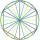Which polygon has 42 more diagonals than sides?
• DiagonalsCalculate the length of the diagonals of the rhombus if its side is long 5 and one of its internal angle is 80°.
• N-gon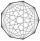How many diagonals has convex 11-gon?
• Regular hexagonal prismCalculate the volume of a regular hexagonal prism whose body diagonals are 24cm and 25cm long.
• Hexagon ABCDEFIn the regular hexagon ABCDEF, the diagonal AE has a length 8cm. Calculate the circumference and the hexagon area.
• Coordinates of the intersection of the diagonals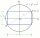In the rectangular coordinate system, a rectangle ABCD is drawn. The vertices of the rectangle are determined by these coordinates A = (2.2) B = (8.2) C = (8.6) D = (2.6) Find the coordinates of the intersection of the diagonals of the ABCD rectangle
• Find the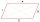Find the content of a regular 12 sided polygon, if its side a = 12 cm.Calculate the content of a regular 15-sides polygon inscribed in a circle with radius r = 4. Express the result to two decimal places.Calculate the size of the smaller of the angles determined by lines A1 A4 and A2 A10 in the regular dodecagon A1A2A3. .. A12. Express the result in degrees.In the diamond ABCD is AB = 4 dm and the length of the diagonal is 6.4 dm long. What is the area of the diamond?